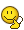# Pendulum with a mass on a container (thermodynamics problem)

#### ValeForce46

Homework Statement
A pendulum, with a block of iron ($m=1 kg$, specific heat $c=448\frac{J}{Kg\cdot K}$) hanging by a thread (mass negligible), is inside a container of volume with rigid and adiabatic walls. Inside the container there's air (to consider as a biatomic ideal gas) at the atmospheric pressure and at the temperature $T_0=300 K$. You observe the pendulum has a speed of $v=3.5 \frac{m}{s}$ when the block is on the vertical. After some time, the pendulum ceases to swing. Determine:
a) The final temperature of the system at the end of the oscillation.
b) The variation of entropy of the universe at the end of the trasformation.
Homework Equations
$Q=m*c*(T_f-T_0)$
The kinetic energy of the pendulum $K=\frac{1}{2}\cdot m\cdot v^2$ will turn into heat (entirely).
So both the air and the block of iron will change their temperature.
To find $n$ (moles of the gas) I can use the ideal gas law:
$n=\frac{pV}{RT}=0.9 mol$
Do I have the following equation?
$\frac{1}{2}mv^2=m*c*(T_f-T_0)+n*c_v*(T_f-T_0)$ and $T_f$ is my unknown.
But I get $T_f=300K$ (nearly). Where's my mistake?

Related Introductory Physics Homework Help News on Phys.org

#### TSny

Homework Helper
Gold Member
To get a rough idea of the change in temperature: How many Joules of KE does the block of iron initially have?

Note that ciron = 448 J/(kg K), which tells you that it takes 448 J of energy to raise the temperature of the iron block by just 1 K.

•ValeForce46

#### ValeForce46

To get a rough idea of the change in temperature: How many Joules of KE does the block of iron initially have?
$K=6.13 J$.
So you're silently (not that much) saying that my result $T_f=300.013 K$ is right?
I even doubted my equation.

#### TSny

Homework Helper
Gold Member
$K=6.13 J$.
So you're silently (not that much) saying that my result $T_f=300.013 K$ is right?
I even doubted my equation.
Yes, I think your answer is correct. I wanted you to see how you can tell that a very small temperature increase is what you would expect.

•ValeForce46

#### ValeForce46

Yeah, you made me realize that. Thanks a lot.
However, for completeness, the variation of entropy of the universe is the variation of entropy of the system, because the walls of the container are adiabatic so there's no variation of entropy of the environment:
$\Delta S_{Universe}=\Delta S_{System}=mc(T_f-T_0)+nc_v(T_f-T_0)=6.1 \frac{J}{K}$

#### TSny

Homework Helper
Gold Member
$\Delta S_{Universe}=\Delta S_{System}=mc(T_f-T_0)+nc_v(T_f-T_0)=6.1 \frac{J}{K}$
Check this calculation. Note that what you calculated here is $\Delta E_{int}$ of the block and gas. It's not surprising that you got 6.1, since the initial KE of the block is 6.1 J.

•ValeForce46

#### ValeForce46

oops.... I did the integral wrong$ΔS_{Universe}=ΔS_{System}=m*c*\ln (\frac{T_f}{T_0})+n*c_v*\ln (\frac{T_f}{T_0})=0.02 \frac{J}{K}$
Hope this is right ....

#### TSny

Homework Helper
Gold Member
oops.... I did the integral wrong$ΔS_{Universe}=ΔS_{System}=m*c*\ln (\frac{T_f}{T_0})+n*c_v*\ln (\frac{T_f}{T_0})=0.02 \frac{J}{K}$
Hope this is right ....
Looks good.

•ValeForce46

#### TSny

Homework Helper
Gold Member
Just a side note: For this problem, you can check that about 96% of the initial KE of the iron block ends up in the iron block and only about 4% goes to the air. That kind of surprised me at first. But it makes sense if you think about the equipartition of energy. In this problem, there are a lot more atoms of iron in the system compared to molecules of air. Moreover, for T around 300 K, each atom of iron has effectively 6 "degrees of freedom" for storing internal energy (Law of Dulong and Petit) compared to 5 degrees of freedom for each air molecule. The energy spreads equally among all of the degrees of freedom in the system.

•ValeForce46

#### Chestermiller

Mentor
The kinetic energy of the pendulum $K=\frac{1}{2}\cdot m\cdot v^2$ will turn into heat (entirely).
This is an incorrect interpretation. The kinetic energy of the pendulum will not turn into heat. There is no heat transferred to this adiabatic system. The correct interpretation starts with the full presentation of the first law of thermodynamics: $$\Delta U+\Delta (KE)+\Delta (PE)=Q-W=0$$Also there is not change in potential energy of the system. So $$\Delta U=-\Delta (KE)$$
This tells us the correct interpretation, namely, that the kinetic energy of the pendulum will convert directly to internal energy (via viscous dissipation by the air).

•ValeForce46 and TSny

#### Chestermiller

Mentor
Since the temperature hardly changes, the change in entropy is nearly exactly the initial kinetic energy (6.125 J) divided by the temperature, roughly (300 K), or 6.125/300 = 0.0204 J/K.

### Want to reply to this thread?

"Pendulum with a mass on a container (thermodynamics problem)"

### Physics Forums Values

We Value Quality
• Topics based on mainstream science
• Proper English grammar and spelling
We Value Civility
• Positive and compassionate attitudes
• Patience while debating
We Value Productivity
• Disciplined to remain on-topic
• Recognition of own weaknesses
• Solo and co-op problem solving